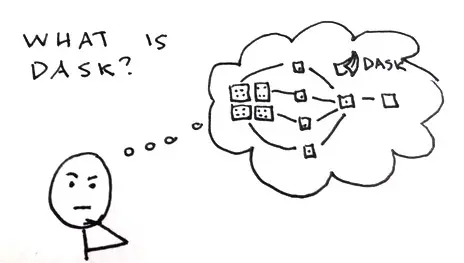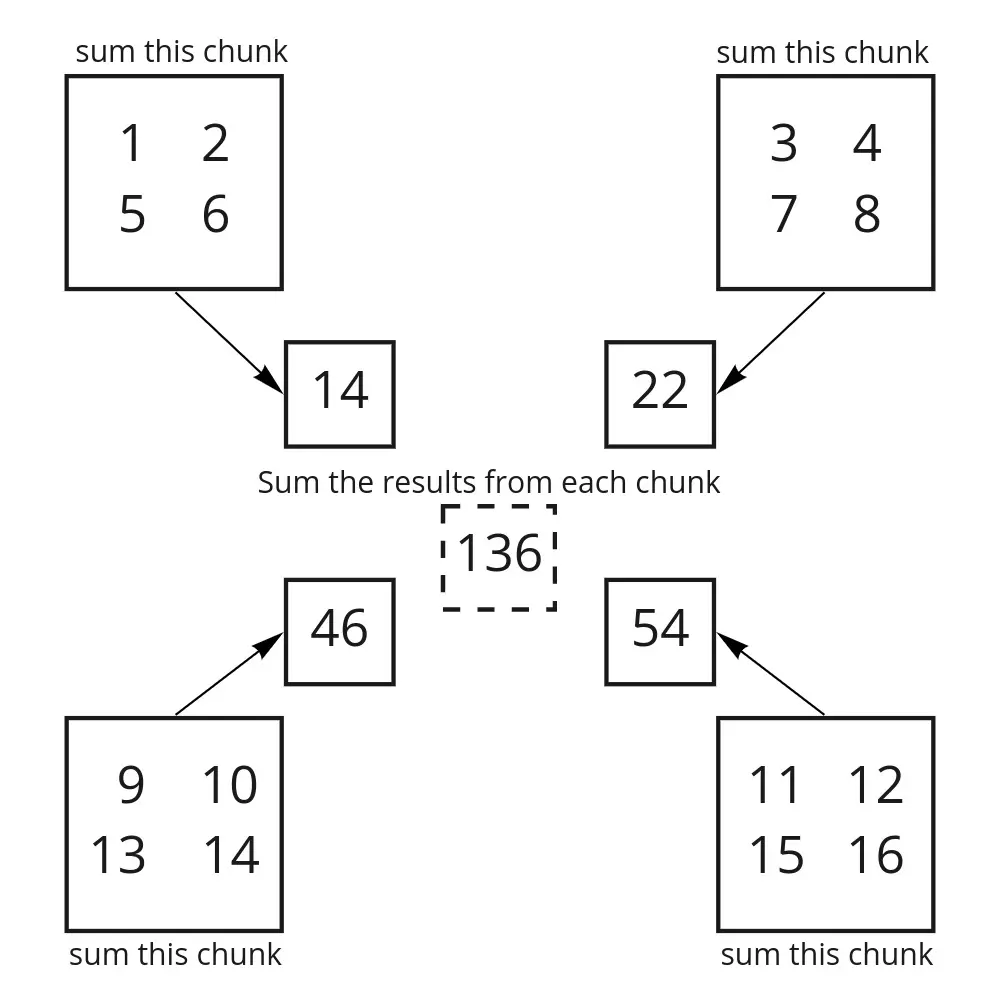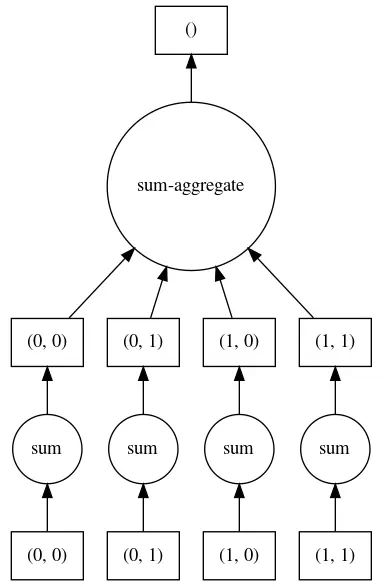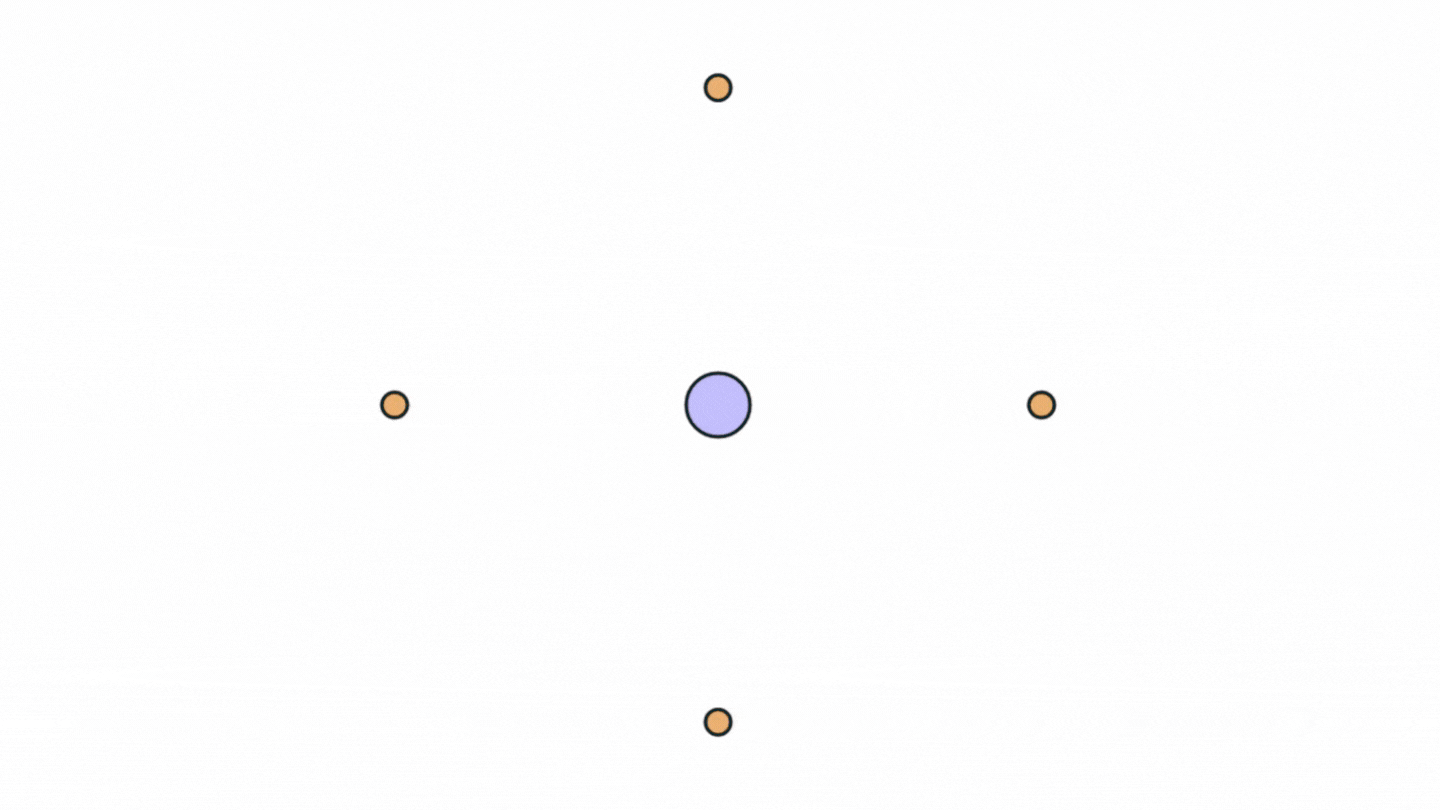# What is Dask and How Does it Work?

The basics of what Dask is, why you’d want to use it, and how to get started.Check out Dask in 15 Minutes by Dan Bochman for a video introduction to Dask

Dask is an open-source Python library that lets you work on arbitrarily large datasets and dramatically increases the speed of your computations. It is available on various data science platforms, including Saturn Cloud.

• Familiar Interface
• Flexibility when you need it
• Python all the way down

## Familiar Interface

Python has a rich ecosystem of data science libraries including numpy for arrays, pandas for dataframes, xarray for nd-data, and scikit-learn for machine learning. Dask matches those libraries.

This means:

• you don’t have to learn a new set of arguments to pass to `read_csv`
• you don’t have to do a massive code-restructure to start using Dask.

#### [Pandas](https://saturncloud.io/glossary/pandas)

``````import pandas as pd
df["profit"].resample("1D").sum()
``````
``````import dask.dataframe as dd
df["profit"].resample("1D").sum()
``````

#### Numpy

``````import numpy.array as np
a = np.array([1, 2, 3, 4])
b = np.arange(5, 9)
a * b
``````
``````import dask.array as da
a = da.array([1, 2, 3, 4])
b = da.arange(5, 9)
a * b
``````

#### xarray

``````import xarray as xr
ds = xr.open_dataset(
"temperature.zarr"
)
ds.air.mean("time")
``````
``````import xarray as xr
ds = xr.open_dataset(
"temperature.zarr",
chunks={"time": 1000}
)
ds.air.mean("time")
``````

## Flexibility when you need it

Sometimes your data doesn’t fit neatly in a dataframe or an array. Or maybe you have already written a whole piece of your pipeline and you just want to make it faster. That’s what `dask.delayed` is for. Wrap any function with the `dask.delayed` decorator and it will run in parallel.

``````import dask

def my_function(x, y):
"""This can do anything you like"""

outputs = []
for x, y in ...:
outputs.append(my_function(x, y))
``````

## Python all the way down

This one is simple. Dask is written in Python and runs Python for people who want to write in Python and troubleshoot in Python.

## How does it work?

There are three main pieces that combine to let Dask effectively run distributed code with minimal effort from you.

• Blockwise algorithms run in parallel on shards of data
• Scheduler decides which worker in a potentially many-node cluster gets which task.

## Blockwise Algorithms

Dask arrays may look like numpy and Dask dataframes may looks pandas, but the actual implementation of each method is rewritten to work in parallel. This means:

• Your data doesn’t have to fit into memory.
• You can operate on different pieces of it at the same time - which is faster.

### How it works

Internally, a Dask array is a bunch of numpy arrays in a particular pattern. Dask implements blockwise operations so that Dask can work on each block of data individually and then combine the results.

Let’s consider a 4x4 array that goes from 1 to 16 and is divided into 4 2x2 chunks.

``````import numpy as np

a = da.from_array(
np.array([
[ 1,  2,  3,  4],
[ 5,  6,  7,  8],
[ 9, 10, 11, 12],
[13, 14, 15, 16]
]),
chunks=(2, 2)
)
``````

When you take the sum of that array (`a.sum()`), Dask first takes the sum of each chunk and only after each of those is completed, takes the sum of the results from each chunk.By having each worker do a sum separately, then combine at the end, Dask is able to do the computation roughly 4 times faster than if there was only a single worker having to do it all on its own.

Dask doesn’t do anything until you tell it to - it’s lazy.

When you call methods - like `a.sum()` - on a Dask object, all Dask does is construct a graph. Calling `.compute()` makes Dask start crunching through the graph.

By waiting until you actually need the answer, Dask gets the chance to optimize the graph. So Dask only ever has to read the data that is needed to get the result that you asked for. By being lazy Dask ends up doing less work! This is often faster than if Dask had to do all the computations the moment the original function is called.

### How it works

Each operation on each block is represented as a task. These tasks are connected together into a graph so that every task knows what has to happen before it runs.

Here is the task graph for the `a.sum()` operation described above.Dask strings together operations by adding layers to the graph. The graph is represented as a dict-like object where each node contains a link to its dependencies.

Right before the graph is computed, there is an optimization step that can consolidate many tasks into one (`fuse`), drop tasks that are no longer needed (`cull`). You can even define your own custom optimizations.

## Scheduler decides who gets what

To each according to their needs… - Karl Marx

Triggering computation on a task graph tells Dask to send the graph to the scheduler. There, each task is assigned to a worker. Depending on how you set things up you might have 4 workers on your personal computer, or you might have 40 workers on an HPC system or on the cloud. The scheduler tries to minimize data transfer and maximize the use of each worker.

This is what it looks like for the scheduler (shown here in purple) to send out the `a.sum()` operation to each of the workers (shown in orange and yellow).Since there are 4 workers, the `sum` on each chunk happens in parallel. This is ultimately what lets Dask be so fast. Each worker only needs to receive a small piece of the data at a time and can operate on it while other workers are operating on the other pieces.

Crucially, this means that the entire dataset is not read by any one worker. So your data can be arbitrarily big and it’s still possible to work with it.

## Conclusion

Sometimes your data is a reasonable size and you can happily use regular numpy and pandas. Other times it isn’t and you can’t. That’s where Dask comes in! Have fun getting started!